Examples

Chapter 3 Class 10 Pair of Linear Equations in Two Variables
Serial order wise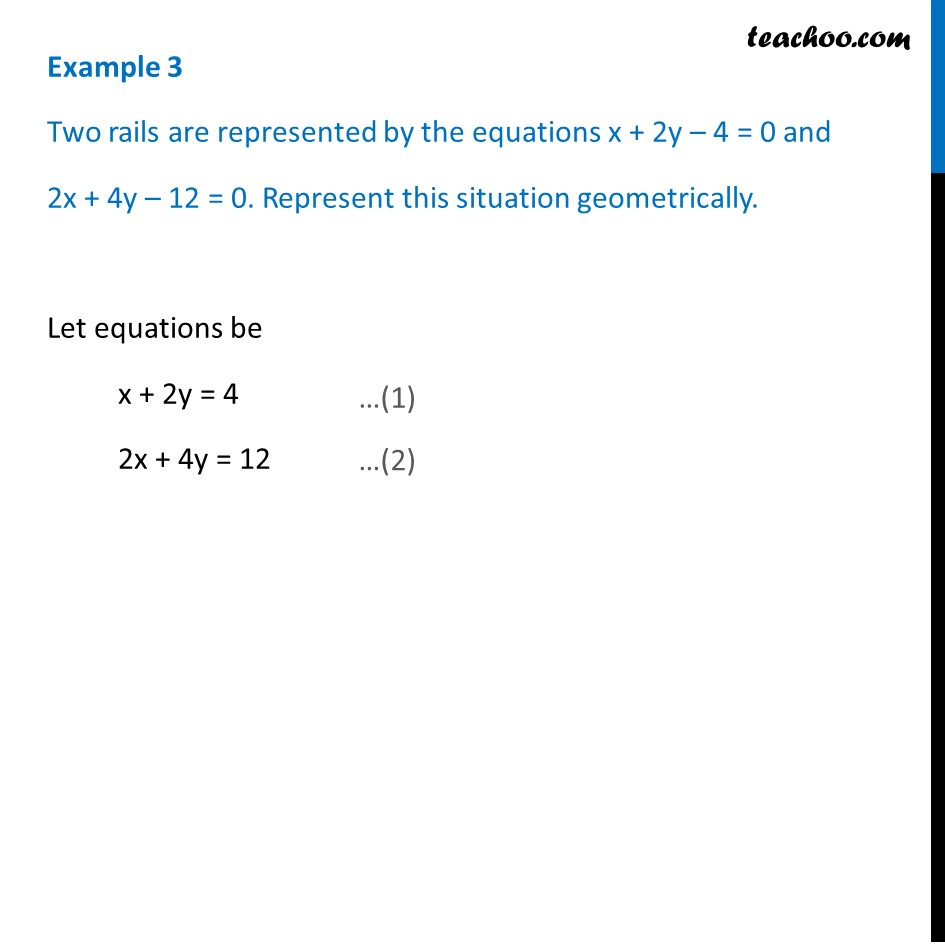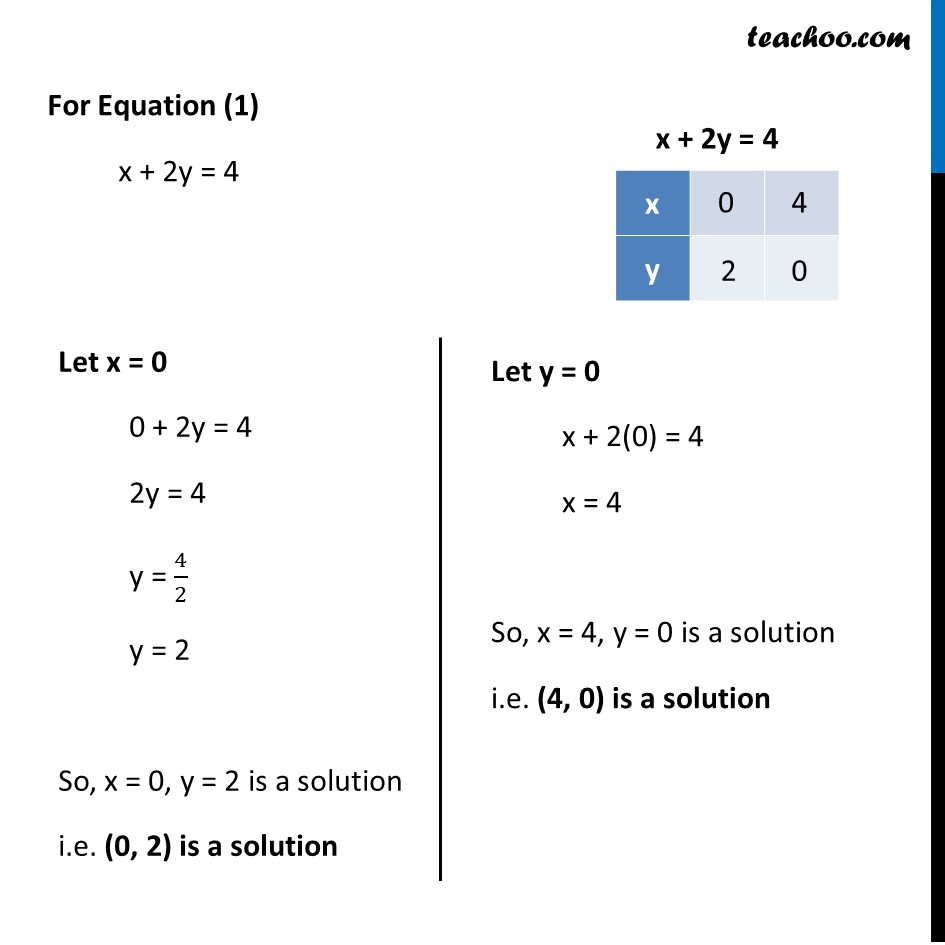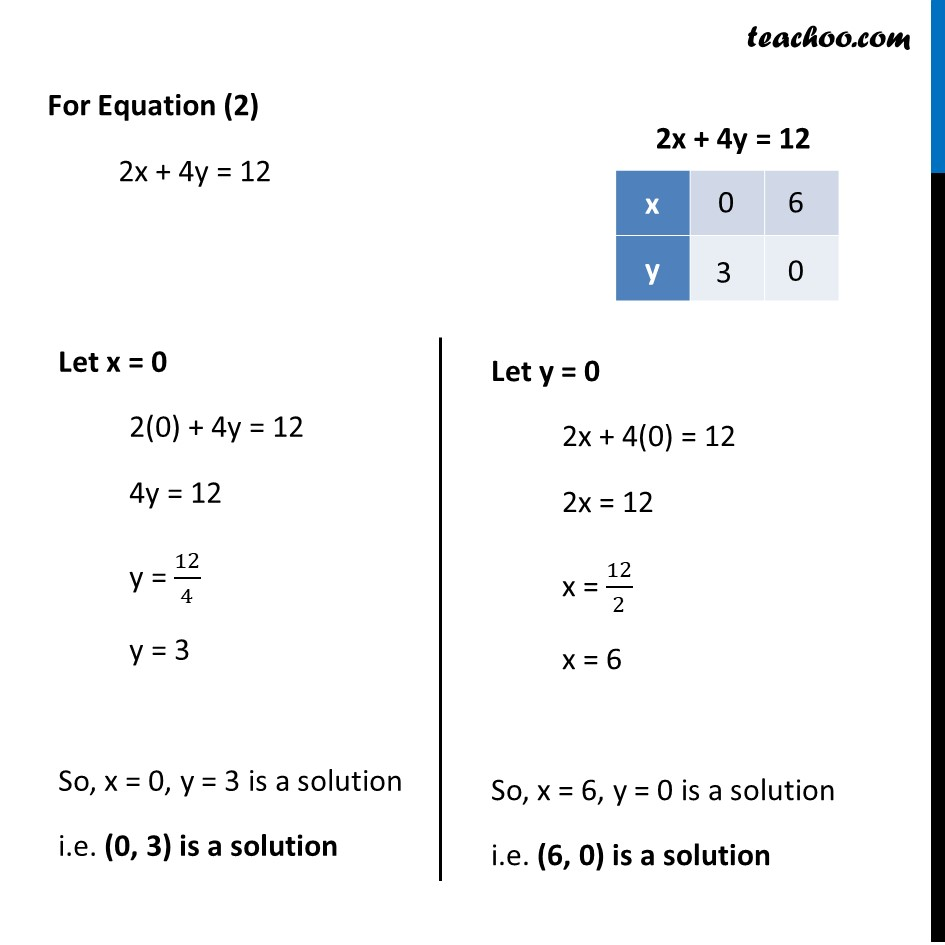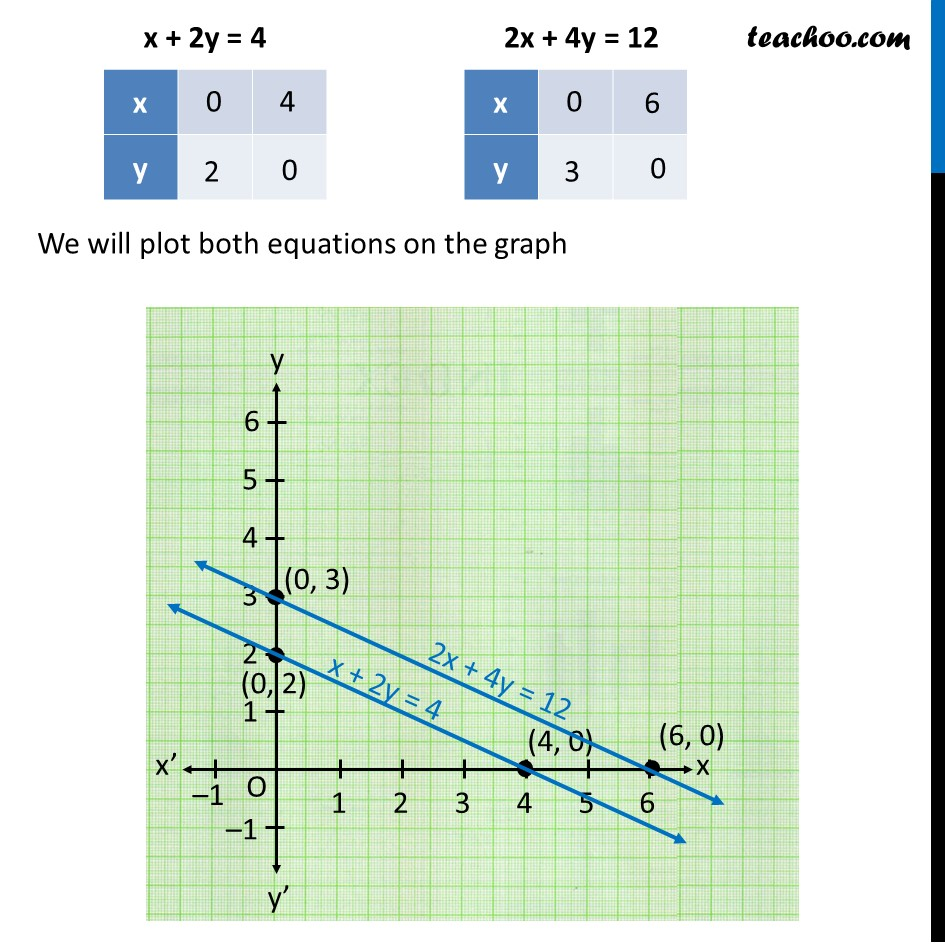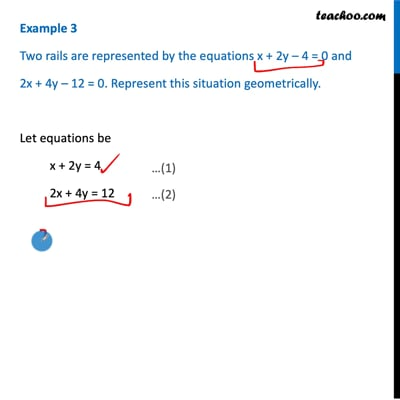This video is only available for Teachoo black users

Solve all your doubts with Teachoo Black (new monthly pack available now!)

### Transcript

Example 3 Two rails are represented by the equations x + 2y – 4 = 0 and 2x + 4y – 12 = 0. Represent this situation geometrically. Let equations be x + 2y = 4 2x + 4y = 12 For Equation (1) x + 2y = 4 Let x = 0 0 + 2y = 4 2y = 4 y = 4/2 y = 2 So, x = 0, y = 2 is a solution i.e. (0, 2) is a solution Let y = 0 x + 2(0) = 4 x = 4 So, x = 4, y = 0 is a solution i.e. (4, 0) is a solution For Equation (2) 2x + 4y = 12 Let x = 0 2(0) + 4y = 12 4y = 12 y = 12/4 y = 3 So, x = 0, y = 3 is a solution i.e. (0, 3) is a solution Let y = 0 2x + 4(0) = 12 2x = 12 x = 12/2 x = 6 So, x = 6, y = 0 is a solution i.e. (6, 0) is a solution We will plot both equations on the graph